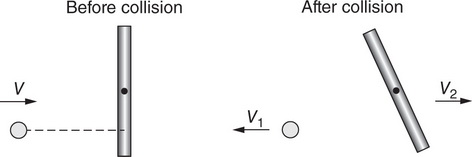# AP Physics 1 Question 177: Answer and Explanation

### Test Information

Question: 177

7.Question below refers to the following information:

A small ball of mass m moving to the right at speed v collides with a stationary rod, as shown in the figure. After the collision, the ball rebounds to the left with speed v1, while the rod’s center of mass moves to the left at speed v2. The rod also rotates counterclockwise.

Is angular momentum about the rod’s center of mass conserved in this collision?

• A. No, the ball always moves in a straight line and thus does not have angular momentum.
• B. No, nothing is spinning clockwise after the collision to cancel the rod’s spin.
• C. Yes, the only torques acting are the ball on the rod and the rod on the ball.
• D. Yes, the rebounding ball means the collision was elastic.